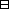## Group Theory and Linear Algebra

Last updated: 27 September 2014

## Lecture 32: Revision: The Fundamental Theorem of Algebra

(II) Week 1 §2: Show that the field of complex numbers is algebraically closed.

To show: If $f={a}_{0}+{a}_{1}t+\cdots +{a}_{\ell }{t}^{\ell }\in ℂ\left[t\right]$ then there exists ${\beta }_{1},{\beta }_{2},\dots ,{\beta }_{\ell }\in ℂ$ such that $a0+a1t+⋯+aℓtℓ =(t-β1)⋯ (t-βℓ).$ Proof by induction on $\ell \text{:}$

If $f={a}_{0}+{a}_{1}t+\cdots +{a}_{\ell }{t}^{\ell }\in ℂ\left[t\right]$ then there exist $\beta \in ℂ$ and ${f}_{1}={b}_{0}+{b}_{1}t+\cdots +{b}_{\ell -1}{t}^{\ell -1}$ such that $f=(t-β)f1.$

Another version of the Lemma is

If $f={a}_{0}+{a}_{1}t+\cdots +{a}_{\ell }{t}^{\ell }\in ℂ\left[t\right]$ then there exist $\beta \in ℂ$ such that $f(β)=0.$

$ℝ$ is not algebraically closed: ${t}^{2}+1$ does not factor in $ℝ\left[t\right],$ even though ${t}^{2}+1=\left(t+i\right)\left(t-i\right)$ factors in $ℂ\left[t\right]\text{.}$

If $f={a}_{0}+{a}_{1}t+\cdots +{a}_{\ell }{t}^{\ell }\in ℝ\left[t\right]$ then there exist ${p}_{1},\dots ,{p}_{r}$ with $\text{deg}\left({p}_{i}\right)$ equal to $1$ or $2$ such that $f=p1·p2⋯pr.$

This follows from the fundamental theorem of algebra and the fact that If $f\in ℝ\left[t\right]$ and $\beta \in ℂ$ such that $f\left(\beta \right)=0$ then $f\left(\stackrel{‾}{\beta }\right)=0\text{.}$ So $f = (t-γ1)⋯(t-γr) (t-β1)(t-β1‾)⋯ (t-βs)(t-βs‾) = (t-γ1)⋯(t-γr) (t2-(β1+β1‾+β1β1‾))⋯ (t2-(βs+βs‾)+βsβs‾)$ with ${\gamma }_{1},\dots ,{\gamma }_{r}\in ℝ$ and ${\beta }_{1},\dots ,{\beta }_{s}\in ℂ$ with ${\beta }_{1},\dots ,{\beta }_{s}\notin ℝ\text{.}$ Note that ${\beta }_{j}+\stackrel{‾}{{\beta }_{j}}\in ℝ$ and ${\beta }_{j}\stackrel{‾}{{\beta }_{j}}\in ℝ\text{.}$

This theorem is called the fundamental theorem of algebra. It was first proved by d'Alembert, after which Gauss studied the theorem intensively providing 14 proofs.

Further references: Wikipedia - Fundamental Theorem of Algebra, Math Overflow - Fundamental Theorem of Algebra, Article of Harm Derksen.

$ℂ$ is algebraically closed.Proof (d'Alembert-Gauss [Bou, Top. Ch VIII §1, no. 1, Theorem 1]).

Bourbaki defines $ℂ$ as $ℝ\left[X\right]\left({X}^{2}+1\right)\text{.}$

To show:
 (a) If $a\in {ℝ}_{\ge 0}$ then there exists $\sqrt{a}\in ℝ\text{.}$ (b) If $p\left(t\right)\in ℝ\left[t\right]$ and $\text{deg} p$ is odd then there exists $\alpha \in ℝ$ such that $p\left(\alpha \right)=0\text{.}$
 (b) Assume $p\left(t\right)={a}_{n}{X}^{n}+{a}_{n-1}{X}^{n-1}+\cdots +{a}_{0}$ with $n$ odd and ${a}_{n}\ne 0\text{.}$ If $x\in ℝ$ and $x\ne 0$ then $p(x)=anxng(x),$ where $g\left(x\right)=1+\frac{{a}_{n-1}}{{a}_{n}x}+\cdots +\frac{{a}_{0}}{{a}_{n}{x}^{n}}\text{.}$ $limx→∞g(x)=1 andlimx→-∞g(x)=1.$ So there exists $a\in {ℝ}_{\ge 0}$ such that $sign(an)= sign(f(a))and sign(-an)= sign(f(-a)).$ Thus, by Bolzano's theorem, [Bou, Top IV §6, no. 1, Theorem 2], there exists $\alpha \in {\left[-a,a\right]}_{ℝ}$ such that $f\left(\alpha \right)=0\text{.}$

$\square$Proof 2 [Bou, Top. Ch VIII §2. Exercise 2]. Let $f\left(t\right)\in ℂ\left[t\right]$ such that $f\left(t\right)\ne 0\text{.}$ To show: There exists $r\in {ℝ}_{\ge 0}$ such that if $z\in ℂ$ and $|z|\ge r$ then $|f\left(z\right)|>|f\left(0\right)|\text{.}$ Use [Exercise 1] and Weierstrass' theorem [Bou, Top. ChIV §6 no. 1, Theorem 1] to show $ℂ$ is algebraically closed. $\square$

[Bou Top. Ch. VIII §2, Exercise 1]
Let $a\in ℂ,$ $a\ne 0$ and $n\in {ℤ}_{>0}\text{.}$

To show:
 (a) If $r\in {ℝ}_{>0}$ such that ${r}^{n}\le |a|$ then there exists $z\in ℂ$ such that $|z|=r$ and $|a+zn|= |a|-rn.$ (b) If $f\left(z\right)\in ℂ\left[t\right]$ and $\text{deg}\left(f\right)>0$ and ${z}_{0}\in ℂ,$ with $f\left({z}_{0}\right)\ne 0$ then there exists $z\in {B}_{\epsilon }\left({z}_{0}\right)$ such that $|f(z0)|> |f(z)|.$

## Notes and References

These are a typed copy of Lecture 32 from a series of handwritten lecture notes for the class Group Theory and Linear Algebra given on October 19, 2011.